omG8HI9LSKFW6lhCj8prTs0Z6lUhdOT9Jhi1Sf4m

# Phasor and Element Circuit Relationship for AC Electric Circuits

In the previous post, we have learned how to write a current or voltage in the frequency or phasor domain. After understand how to convert the current or voltage in time domain to frequency domain or vice versa, now we will learn how to implement it to circuits consisting of elements R, L, and C.
Make sure to read what is ac circuit first.

## Phasor Relationship for Circuit Elements

What we need to do first is to convert the voltage-current relationship from the time domain to the frequency domain for every element. Passive sign convention will still be used in this process. For a starter, we will use the resistor. Assume the current flowing through a resistor R is i = Im cos(ωt + ), then the voltage across it with Ohm's law is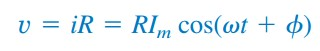(1)
Its phasor form of the voltage is(2)
But the phasor representation of the current is IIm∠∅. Thus,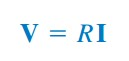(3)
it shows us that the voltage-current relationship for a resistor in the phasor domain continues to be Ohm's law, as in the time domain. Figure.(1) draws the voltage-current relationship of a resistor.Figure 1. Relationship of voltage-current for a resistor in the : (a) time domain, (b) frequency domain
We should notice from Equation.(3) that the voltage and current are in phase, as drawn in the phasor diagram of Figure.(2).Figure 2. Phasor diagram for the resistor
Now we try with inductor L, assume the current through it is i = Im cos(ωt + ). The voltage across the inductor is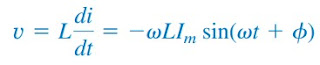(4)
Remember from the previous post about sinusoidal, that -sin A = cos(A + 90o). We can rewrite the voltage as(7)
showing that the voltage has a magnitude of ωLIm and a phase of  + 90o. The voltage and current are 90o out of phase. Specifically, the current lags the voltage by 90o. Figure.(3) draws the voltage-current relations for the inductor and the Figure.(4) draws the phasor diagram.Figure 3. Relationship of voltage-current for an inductor in the : (a) time domain, (b) frequency domainFigure 4. Phasor diagram for the inductor; I lags V
Move on to capacitor C, assume the voltage across it is v = Vm cos(ωt + ). Its flowing current through the capacitor is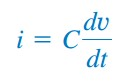(8)
Using the previous steps, we apply the transformation of derivative v(t) to the phasor domain as jωV as in here (Equation.15) and Equation.(8) above to obtain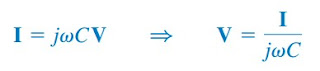(9)
and shows us that the current and voltage are 90o out of phase. To be more precise, the current leads the voltage by 90o.Figure 5. Relationship of voltage-current for a capacitor in the: (a) time domain, (b) frequency domain
From Figure.(5) we notice the relationship of voltage-current for a capacitor.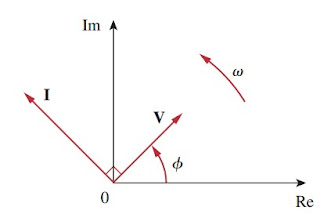Figure 6. Phasor diagram for the capacitor ; I leads V
Figure.(6) shows the phasor diagram of the capacitor. Table.(1) gives a brief summary of the time domain and phasor domain representative of the circuit elements.Table 1

## Phasor Relationship for Circuit Elements Example

For a better understanding let us review the example below :
1. Voltage v = 12 cos(60t + 45o) is applied to an inductor with 0.1 H. Find the steady-state current through the inductor.
Solution :
For the inductor, V = jωLI, where ω = 60 rad/s and V = 1245o V. Thus,
Let us convert the result above and we get the time domain form,

Have you understood what is phasor relationship with elements? Don't forget to share and subscribe! Happy learning!
Reference:  Fundamentals of electric circuits by Charles K. Alexander and Matthew N. O. Sadiku

Untuk Bahasa Indonesia baca Hubungan Fasor dan Elemen Rangkaian.
Related Posts
SHARE